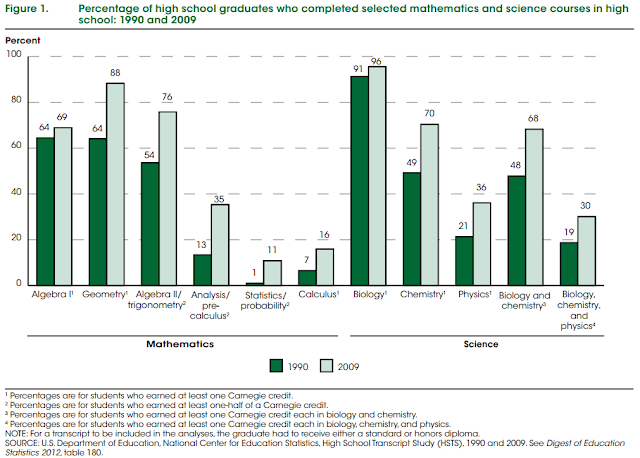### American High Schools Are Taking More Advanced Courses in Science and Math

Caralee Adams in Education Week writes "High School Students Taking More Math and Science Courses":
"High school students are being told to take more rigorous math and science courses if they want to be prepared for college and get lucrative jobs in STEM careers.
New data from the National Center for Education Statistics suggest they are taking that advice."
The data Adams is referring to is summarized in the graph below:Above figure captured from http://nces.ed.gov/pubs2013/2013037.pdf
To understand what the above numbers mean, the following pieces of information are important:

First, what is a Carnegie credit? The most widely used credit systems in U.S. secondary education are based on the Carnegie Unit system. Carnegie Units were proposed in 1906 as a basis for measuring school work. A unit would represent a single subject taught for one classroom period for five days a week.(Structure of the U.S. Education System: Credit Systems, U.S. Department of Education, 2008)

Second, what qualifies as an advanced course in math or science? To answer this question, one must get acquainted first with "pipeline measures". The following are pertinent descriptions of what these measures entail:
(http://nces.ed.gov/pubs2007/2007312.pdf)

The pipeline measures are built on the assumption that students who complete advanced level coursework have mastered the skills of lower level courses and gained experience with more complex and advanced subject matter. In turn, these students should have a greater understanding of the subject area than their peers who reach only lower levels of the pipeline.
The categories for math are as follows:
• The no mathematics or low academic mathematics course level included − 1 = no mathematics or low academic mathematics (other general mathematics, mathematics 7, accelerated mathematics 7, mathematics 8, accelerated mathematics 8, general mathematics 1, general mathematics 2, science mathematics, mathematics in the arts, vocational mathematics, technical mathematics, mathematics review, mathematics tutoring, consumer mathematics, other actuarial sciences, other applied mathematics, basic mathematics 1, basic mathematics 2, basic mathematics 3, basic mathematics 4, pre-algebra, algebra I–part 1, algebra I–part 2, or informal geometry).
• Middle academic mathematics course levels included
− 2 = algebra I/plane geometry (other pure mathematics, algebra I, plane geometry, solid geometry, unified mathematics 1, unified mathematics 2, geometry–part 1, geometry–part 2, unified mathematics 1–part 1, unified mathematics 1–part 2, pre-International Baccalaureate [IB] geometry, IB mathematics methods 1, IB mathematics studies 1, discrete mathematics, finite mathematics, algebra and geometry, or other mathematics);
− 3 = algebra II (algebra II, unified mathematics III, or pre-IB algebra II/ trigonometry); and
− 4 = algebra III/trigonometry/analytic geometry (algebra III, trigonometry, analytic geometry, trigonometry and solid geometry, algebra and trigonometry, algebra and analytic geometry, linear algebra, independent study, statistics, probability, probability and statistics, or Advanced Placement [AP] statistics). • Advanced academic mathematics course levels included
− 5 = precalculus (introductory analysis or IB mathematics studies 2); and
− 6 = calculus (calculus and analytic geometry, calculus, AP calculus, IB mathematics studies/calculus, or AP calculus CD).
The categories for science are as follows:
• 1 = no science or low academic science (unified science, physical science, applied physical science, other geological sciences, earth science, college preparatory earth science, or miscellaneous physical sciences);
• 2 = secondary physical science and basic biology (basic biology 1, basic biology 2, other biological and physical sciences, independent science study, outdoor education, other systems science, futuristics, environmental science, IB environmental studies, energy and environment, astronomy, other astronomy, other astrophysics, atmospheric sciences and meteorology, meteorology, introductory chemistry, chemistry in the community, organic biochemistry, physical chemistry, consumer chemistry, chemistry independent study, other chemistry, AP environmental science, geology, geology–field studies, mineralogy, oceanography, general physics, other physics, electricity and electronics science, acoustics, other planetary science, other rocketry and space science, or aerospace science);
• 3 = general biology (general biology 1, general biology 2, honors biology 1, other biology, pre-IB biology, ecology, marine biology, advanced marine biology, zoology, other zoology, vertebrate zoology, invertebrate zoology, animal behavior, human physiology, advanced physiology, pathology, comparative embryology, or other life sciences);
• 4 = chemistry I or physics I (chemistry 1, pre-IB chemistry, or physics 1);
• 5 = chemistry I and physics I (highest completed courses include one level I chemistry course [see above] and one level I physics course [see above]); and
• 6 = chemistry II or physics II or advanced biology (chemistry 2, IB chemistry 2, IB chemistry 3, AP chemistry, physics 2, IB physics, AP physics B, AP physics C: mechanics, AP physics C: electricity/magnetism, physics 2 without calculus, advanced biology, field biology, genetics, biopsychology, biology seminar, biochemistry and biophysics, biochemistry, botany, other botany, cell and molecular biology, cell biology, microbiology, microbiology other, other life sciences specialized areas, anatomy, IB biology 2, IB biology 3, or AP biology).